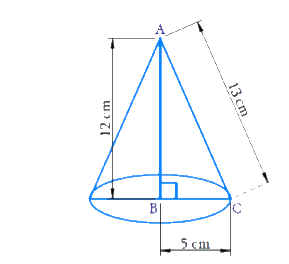# Ex.13.7 Q7 Surface Areas and Volumes Solution - NCERT Maths Class 9

Go back to  'Ex.13.7'

## Question

A right triangle $$ABC$$ with sides $$5\rm\, cm,$$ $$12 \rm\,cm$$ and $$13\rm\, cm$$ is revolved about the side $$12 \rm\,cm.$$ Find the volume of the solid so obtained.

Video Solution
Surface-Areas-And-Volumes
Ex exercise-13-7 | Question 7

## Text Solution

Reasoning:

Volume of the cone is \begin{align}\frac{1}{3} \end{align} times of the volume of a cylinder \begin{align} = \frac{1}{3}\pi {r^2}h \end{align}.

What is known?

Sides of the right triangle.

What is unknown?

Volume of the solid obtained.

Steps:Radius $$(r) = 5\rm\, cm$$

height $$(h) = 12 \rm\,cm$$

Volume of cone

\begin{align}&= \frac{1}{3}\pi {r^2}h \\ &= \frac{1}{3} \times \frac{{22}}{7} \times 5 \times 5 \times 12\\ &= \frac{{2200}}{7}\,\,or\,\,100\pi \end{align}

Volume of the cone \begin{align}100\pi \,\,\rm\,c{m^3} \end{align}.